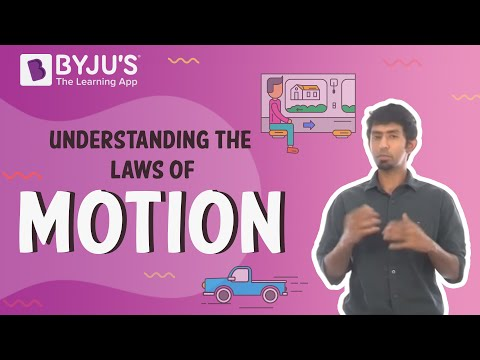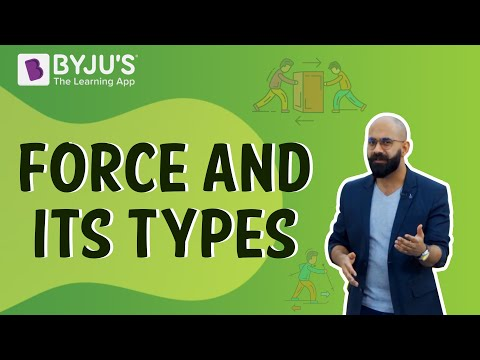# Balanced Force Questions

## Balanced Forces

Balanced forces are the forces that are identical in size and opposite in direction. When the forces are balanced, there exists no change in motion.

A force is nothing but just a push or pull on an object measured in newtons (N). And if the forces are balanced, it means that any pushes and pulls are balanced by another force in the opposite direction in every single direction. So, for example,

Imagine you are playing a game of tug-of-war with your friends. Your team starts by pulling really hard, with all its strength. But, in the opposite direction, the other team is also pulling with equal force. The flag in the centre of the rope does not move. The flag does not move because the force applied by the other team is equal to the force your team is pulling in the opposite direction. Since these forces are in opposite directions, it means that these forces on the rope are balanced. Therefore, when the forces are balanced, the flag in the centre of the rope will not move and to win the game, any team needs to apply more force than the other.

### Characteristics of Balanced Force –

• Balanced forces have equal size and equal magnitude.
• Balanced forces work in opposite directions on an object.
• The state of rest or motion of an object doesn’t change in balanced forces.
• Due to balanced forces, an object in motion cannot change its direction or speed, and also the net forces acting are zero in balanced forces.

### Important Questions on Balanced Forces

1) If a force of 40 N is applied to the body on the left side while a force of 40N is applied to the body on the right side, and at first the body is at rest. What will be the behaviour of the motion of the body?

The body will be at rest because the net force on the body is 0N, which means that there is no change in the state of motion of the body.

2) Define balanced and unbalanced forces.

Balanced forces: Balanced forces are the forces which when acted on a body do not change their state of rest or state of uniform motion.

Unbalanced forces: When the resultant force acting on a body is not equal to zero, and which eventually results in the motion of the body, such forces are called unbalanced forces.

3) What are the characteristics of balanced forces?

The following points are some important characteristics of balanced forces-

• Balanced forces have equal size and equal magnitude.
• Balanced forces work in opposite directions on an object.
• The state of rest or motion of an object doesn’t change in balanced forces.
• Due to balanced forces, an object in motion cannot change its direction or speed, and also the net forces acting are zero in balanced forces.

4) What are balanced forces equivalent to?

As per the definition, the forces are said to be balanced if equal forces are acting in opposite directions. This means that the combined forces that are having a balance equal to zero are termed as balanced forces equivalent. Therefore, the balanced forces are equivalent to no force at all.

5) How will you determine whether a force is a balanced force or not?

To determine whether the force acting upon the object is balanced, we need to analyse what forces are acting upon the object and in what direction. If two individual forces are acting in opposite directions and are equal in magnitude, then the force is said to be balanced.

6) A cyclist travelling at a speed of 20m/s has a forward force of 300N but is acted on by air and friction resistance of 300N which pushes against her. What will happen to the cyclist’s motion?

The force exerted by the cyclist and the force acted on her, that is the air and friction resistance, are both equal and opposite to each other. Hence, these forces are balanced, cancelling each other out, and therefore, there will be no change in the motion and the cyclist will continue to move at a constant speed of 20m/s

7) Give 3 examples of balanced forces.

• An object floating in water
• A jacket hanging on a hook
• Planets orbiting around the sun in fixed orbits

8) Give 3 examples of unbalanced forces.

• An object sinking in water
• A fruit dropping from a tree
• A group of kids winning a tug of war game

9) State Newton’s first Law of Motion.

Newton’s first law states that, a body at rest will remain at rest, a body in motion will remain in motion until and unless some external unbalanced force is applied on it.

10) Floating of objects is an example of balanced force, explain.

Consider an example of a log floating in the pool of water. The log floats because its weight is balanced by the uprising from the water. This uprising force acts as an equal and opposite force of same magnitude that resists the log from sinking down. Therefore, due to this reason the log floats on the surface of water.

### Practice Questions

1. Differentiate between balanced and unbalanced force.
2. Define balanced forces.
3. What happens when forces are balanced?
4. State and explain Newton’s Laws of Motion.
5. Give 5 examples of balanced forces.
 Related Articles

## Suggested VideosStay tuned to BYJU’S and Fall in Love with Learning!